# imshow

## Syntax

``imshow(I)``
``imshow(I,[low high])``
``imshow(I,[])``
``imshow(RGB)``
``imshow(BW)``
``imshow(X,map)``
``imshow(filename)``
``imshow(___,Name,Value)``
``himage = imshow(___)``
``imshow(I,RI)``
``imshow(X,RX,map)``

## Description

example

````imshow(I)` displays the grayscale image `I` in a figure. `imshow` uses the default display range for the image data type and optimizes figure, axes, and image object properties for image display.```
````imshow(I,[low high])` displays the grayscale image `I`, specifying the display range as a two-element vector, `[low high]`. For more information, see the `DisplayRange` parameter.```

example

````imshow(I,[])` displays the grayscale image `I`, scaling the display based on the range of pixel values in `I`. `imshow` uses `[min(I(:)) max(I(:))]` as the display range. `imshow` displays the minimum value in `I` as black and the maximum value as white. For more information, see the `DisplayRange` parameter.```

example

````imshow(RGB)` displays the truecolor image `RGB` in a figure.```

example

````imshow(BW)` displays the binary image `BW` in a figure. For binary images, `imshow` displays pixels with the value `0` (zero) as black and `1` as white.```

example

````imshow(X,map)` displays the indexed image `X` with the colormap `map`. A colormap matrix can have any number of rows, but it must have exactly 3 columns. Each row is interpreted as a color, with the first element specifying the intensity of red, the second green, and the third blue. Color intensity can be specified on the interval [0, 1].```

example

````imshow(filename)` displays the image stored in the graphics file specified by `filename`.```
````imshow(___,Name,Value)` displays an image, using name-value pairs to control aspects of the operation. ```
````himage = imshow(___)` returns the image object created by `imshow`.```
````imshow(I,RI)` displays the image `I` with associated 2-D spatial referencing object `RI`.```
````imshow(X,RX,map)` displays the indexed image `X` with associated 2-D spatial referencing object `RX` and colormap `map`.```

## Examples

collapse all

Display a grayscale, RGB (truecolor), indexed or binary image using `imshow`. MATLAB® includes a TIF file, named `corn.tif`, that contains three images: a grayscale image, an indexed image, and a truecolor (RGB) image. This example creates a binary image from the grayscale image.

Display a Grayscale Image

Read the grayscale image from the `corn.tif` file into the MATLAB workspace. The grayscale version of the image is the third image in the file.

`corn_gray = imread('corn.tif',3);`

Display the grayscale image using `imshow`.

`imshow(corn_gray)`Display an Indexed Image

Read the indexed image from the `corn.tif` file into the MATLAB workspace. The indexed version of the image is the first image in the file.

`[corn_indexed,map] = imread('corn.tif',1);`

Display the indexed image using `imshow`.

`imshow(corn_indexed,map)`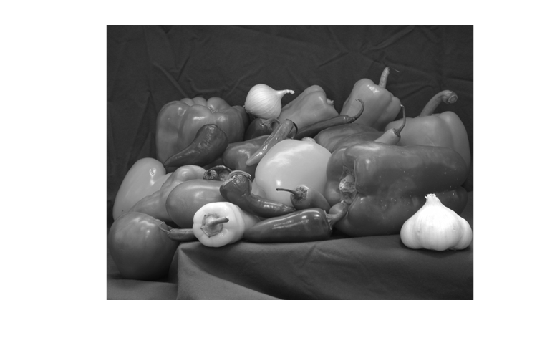Display an RGB Image

Read the RGB image from the `corn.tif` file into the MATLAB workspace. The RGB version of the image is the second image in the file.

`[corn_rgb] = imread('corn.tif',2);`

Display the RGB image using `imshow`.

`imshow(corn_rgb)`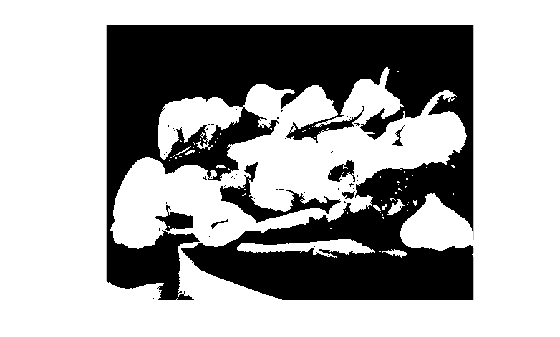Display a Binary Image

Read the grayscale image from the `corn.tif` file into the MATLAB workspace and use thresholding to convert it into a binary image. The grayscale version of the image is the third image in the file.

`[corn_gray] = imread('corn.tif',3);`

Determine the mean value of pixels in the grayscale image.

`meanIntensity = mean(corn_gray(:));`

Create a binary image by thresholding, using the mean intensity value as the threshold.

`corn_binary = corn_gray > meanIntensity;`

Display the binary image using `imshow`.

`imshow(corn_binary)`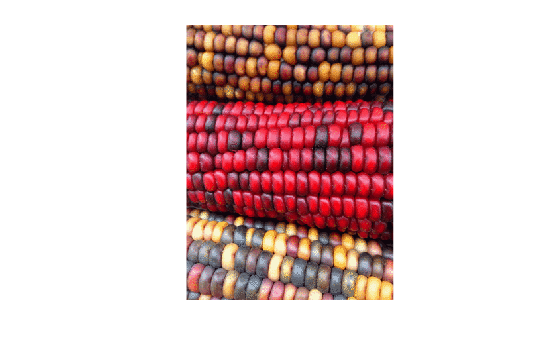Display an image stored in a file.

`imshow('peppers.png');`Read a sample indexed image, `corn.tif`, into the workspace, and then display it.

```[X,map] = imread('corn.tif'); imshow(X,map)```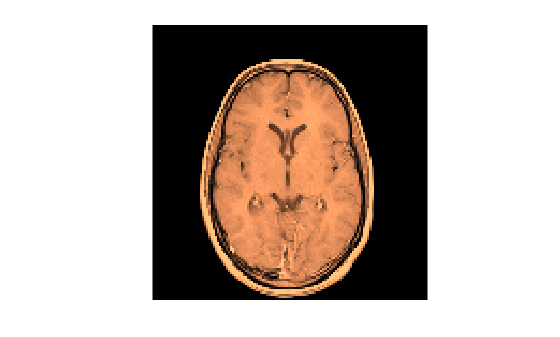Change the colormap for the image using the `colormap` function and specifying the target axes as the first input argument. Use the original colormap without the red component.

```newmap = map; newmap(:,1) = 0; colormap(gca,newmap)```Read a truecolor (RGB) image into the workspace. The data type of the image is `uint8`.

`RGB = imread('peppers.png');`

Extract the green channel of the image. The green channel is the second color plane.

```G = RGB(:,:,2); imshow(G)```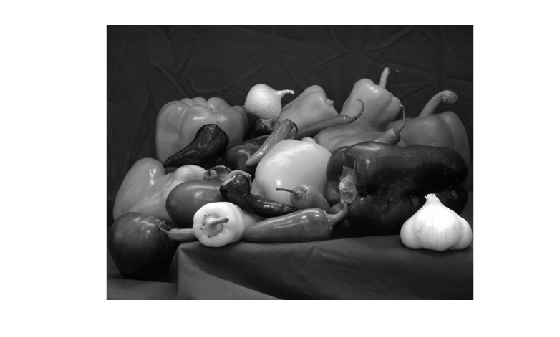Create a filter that blurs the image.

`smoothing_filter = ones(5);`

Filter the green channel of the image using the `filter2` function. The result is an image of data type `double`. The minimum pixel value is 165 and the maximum pixel value is 6,371.

`J = filter2(smoothing_filter,G);`

Display the filtered image using `imshow` with the default display range. For images of data type `double`, the default display range is [0, 1]. The image appears entirely white because all pixel values exceed the maximum of the display range.

`imshow(J)`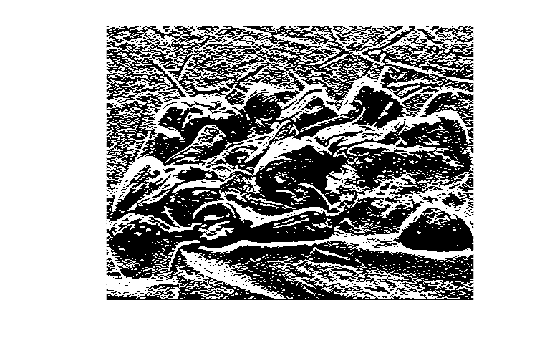Display the filtered image and scale the display range to the pixel values in the image. The image displays with the full range of grayscale values.

`imshow(J,[])`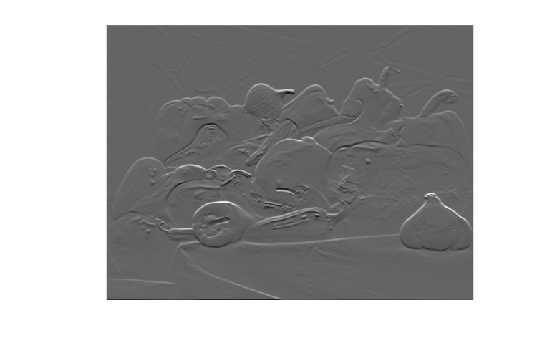Read the grayscale image from the `corn.tif` file into the workspace. The grayscale version of the image is the second image in the file.

`corn_gray = imread('corn.tif',2);`

Select a small portion of the image. Display the detail image at 100% magnification using `imshow`.

```corn_detail = corn_gray(1:100,1:100); imshow(corn_detail)```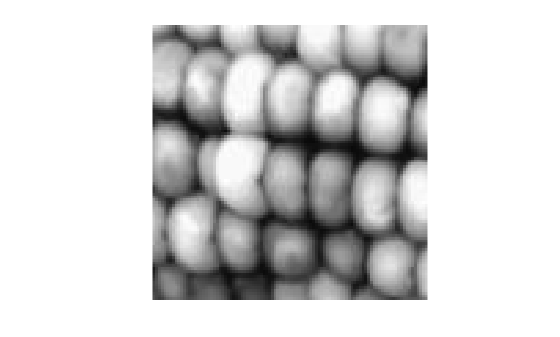Display the image at 1000% magnification by using the `'InitialMagnification'` name-value pair argument. By default, `inshow` performs nearest neighbor interpolation of pixel values. The image has blocking artifacts.

`imshow(corn_detail,'InitialMagnification',1000)`Display the image at 1000% magnification, specifying the bilinear interpolation technique. The image appears smoother.

`imshow(corn_detail,'InitialMagnification',1000,'Interpolation',"bilinear")`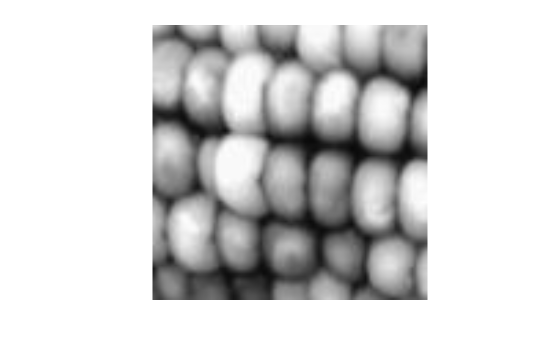`I = imread('pout.tif');`

Display the image. Note the axes limits reflect the size of the image.

```figure imshow(I)```Create a spatial referencing object associated with the image. Use the referencing object to set the x- and y-axes limits in the world coordinate system.

```RI = imref2d(size(I)); RI.XWorldLimits = [0 3]; RI.YWorldLimits = [2 5];```

Display the image, specifying the spatial referencing object. Note the change to the x- and y-axes limits.

```figure imshow(I,RI)```## Input Arguments

collapse all

Input grayscale image, specified as a matrix. A grayscale image can be any numeric data type.

Data Types: `single` | `double` | `int8` | `int16` | `int32` | `int64` | `uint8` | `uint16` | `uint32` | `uint64` | `logical`

Input truecolor image, specified as an m-by-n-by-3 array.

If you specify a truecolor image of data type `single` or `double`, then values should be in the range [0, 1]. If pixel values are outside this range, then you can use the `rescale` function to scale pixel values to the range [0, 1]. The `'DisplayRange'` argument has no effect when the input image is truecolor.

Data Types: `single` | `double` | `uint8` | `uint16`

Input binary image, specified as a matrix.

Data Types: `logical`

Indexed image, specified as a 2-D array of real numeric values. The values in `X` are indices into the colormap specified by `map`.

Data Types: `single` | `double` | `uint8` | `logical`

Colormap, specified as an c-by-3 array of type `single` or `double` in the range `[0 1]`, or a c-by-3 array of type `uint8`. Each row specifies an RGB color value.

Data Types: `single` | `double` | `uint8`

File name, specified as a character vector. The image must be readable by `imread`. The `imshow` function displays the image, but does not store the image data in the MATLAB® workspace. If the file contains multiple images, `imshow` displays the first image in the file.

Example: `imshow('peppers.png')`

Data Types: `char`

Grayscale image display range, specified as a two-element vector. For more information, see the `'DisplayRange'` name-value pair argument.

Example: `[50 250]`

Data Types: `single` | `double` | `int8` | `int16` | `int32` | `int64` | `uint8` | `uint16` | `uint32` | `uint64`

2-D spatial referencing object associated with input image, specified as an `imref2d` object.

2-D spatial referencing object associated with an indexed image, specified as a `imref2d` object.

### Name-Value Pair Arguments

Specify optional comma-separated pairs of `Name,Value` arguments. `Name` is the argument name and `Value` is the corresponding value. `Name` must appear inside quotes. You can specify several name and value pair arguments in any order as `Name1,Value1,...,NameN,ValueN`.

Example: `imshow('board.tif','Border','tight')`

Figure window border space, specified as the comma-separated pair consisting of `'Border'` and either `'tight'` or `'loose'`. When set to `'loose'`, the figure window includes space around the image in the figure. When set to `'tight'`, the figure window does not include any space around the image in the figure.

If the image is very small or if the figure contains other objects besides an image and its axes, `imshow` might use a border regardless of how this parameter is set.

Example: `imshow('board.tif','Border','tight')`

Data Types: `char`

Colormap, specified as the comma-separated pair consisting of `'Colormap'` and a c-by-3 matrix with values in the range [0, 1]. `imshow` uses this to set the colormap for the axes. Use this parameter to view grayscale images in false color. If you specify an empty colormap (`[]`), then `imshow` ignores this parameter.

Note

Starting in R2016b, `imshow` changes the colormap for the axes that contains the image instead of the figure.

Example: ```newmap = copper; imshow('board.tif','Colormap',newmap)```

Data Types: `double`

Display range of a grayscale image, specified as a two-element vector of the form `[low high]`. The `imshow` function displays the value `low` (and any value less than `low`) as black, and it displays the value `high` (and any value greater than `high`) as white. Values between `low` and `high` are displayed as intermediate shades of gray, using the default number of gray levels.

If you specify an empty matrix (`[]`), then `imshow` uses a display range of `[min(I(:)) max(I(:))]`. In other words, the minimum value in `I` is black, and the maximum value is white.

If you do not specify a display range, then `imshow` selects a default display range based on the image data type.

• If `I` is an integer type, then `DisplayRange` defaults to the minimum and maximum representable values for that integer class. For example, the default display range for `uint16` arrays is [0, 65535].

• If `I` is data type `single` or `double`, then the default display range is [0, 1].

Note

Including the parameter name is optional, except when the image is specified by a file name. The syntax ```imshow(I,[low high])``` is equivalent to `imshow(I,'DisplayRange',[low high])`. If you call `imshow` with a file name, then you must specify the `'DisplayRange'` parameter.

Example: `imshow(I,'DisplayRange',[0 80]);`

Data Types: `single` | `double` | `int8` | `int16` | `int32` | `int64` | `uint8` | `uint16` | `uint32` | `uint64`

Initial magnification of image display, specified as the comma-separated pair consisting of `'InitialMagnification'` and a numeric scalar or `'fit'`. If set to `100`, then `imshow` displays the image at 100% magnification (one screen pixel for each image pixel). If set to `'fit'`, then `imshow` scales the entire image to fit in the window.

Initially, `imshow` attempts to display the entire image at the specified magnification. If the magnification value is so large that the image is too big to display on the screen, `imshow` displays the image at the largest magnification that fits on the screen.

If the image is displayed in a figure with its `'WindowStyle'` property set to `'docked'`, then `imshow` displays the image at the largest magnification that fits in the figure.

Note: If you specify the axes position, `imshow` ignores any initial magnification you might have specified and defaults to the `'fit'` behavior.

When you use `imshow` with the `'Reduce'` parameter, the initial magnification must be `'fit'`.

In MATLAB Online™, `'InitialMagnification'` is set to `'fit'` and cannot be changed.

Example: `h = imshow(I,'InitialMagnification','fit');`

Data Types: `single` | `double` | `int8` | `int16` | `int32` | `int64` | `uint8` | `uint16` | `uint32` | `uint64` | `char`

Interpolation technique used when scaling an image, specified as the comma-separated pair consisting of `'Interpolation'` and one of these values.

ValueDescription
`'nearest'`Nearest neighbor interpolation (default). The value of a pixel located at (x, y) is the value of the pixel that is closest to (x, y) in the original image.
`'bilinear'`Bilinear interpolation. The value of a pixel located at (x, y) is a weighted average of the surrounding pixels in the original image.

The interpolation technique applies to the initial magnification and when zooming in or out of the displayed image.

Parent axes of image object, specified as the comma-separated pair consisting of `'Parent'` and an `Axes` object or a `UIAxes` object. Use the `'Parent'` name-value argument to build a UI that gives you control of the `Figure` and `Axes` properties.

Indicator for subsampling image, specified as the comma-separated pair consisting of `'Reduce'` and either `true`, `false`, `1`, or `0`. This argument is valid only when you use it with the name of a TIFF file. Use the `Reduce` argument to display overviews of very large images.

Data Types: `logical`

X-axis limits of nondefault coordinate system, specified as the comma-separated pair consisting of `'XData'` and a two-element vector. This argument establishes a nondefault spatial coordinate system by specifying the image `XData`. The value can have more than two elements, but `imshow` uses only the first and last elements.

Example: `'XData',[100 200]`

Data Types: `single` | `double` | `int8` | `int16` | `int32` | `int64` | `uint8` | `uint16` | `uint32` | `uint64`

Y-axis limits of nondefault coordinate system, specified as the comma-separated pair consisting of `'YData'` and a two-element vector. The value can have more than two elements, but `imshow` uses only the first and last elements.

Example: `'YData',[100 200]`

Data Types: `single` | `double` | `int8` | `int16` | `int32` | `int64` | `uint8` | `uint16` | `uint32` | `uint64`

## Output Arguments

collapse all

Image created by `imshow`, specified as an image object.

## Tips

• To change the colormap after you create the image, use the `colormap` command. Specify the axes that contains the image as the first input argument and the colormap you want as the second input argument. For an example, see Change Colormap of Displayed Image.

• You can display multiple images with different colormaps in the same figure using `imshow` with the `tiledlayout` and `nexttile` functions.

• You can create an axes on top of the axes created by `imshow` by using the `hold on` command after calling `imshow`.

• If you have Image Processing Toolbox™, then you can use the Image Viewer app as an integrated environment for displaying images and performing common image processing tasks.

• If you have Image Processing Toolbox, then you can set toolbox preferences that modify the behavior of `imshow` by using the `iptsetpref` function.

• The `imshow` function is not supported when you start MATLAB with the `-nojvm` option.